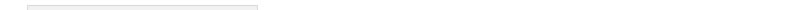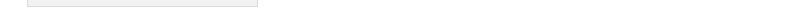Sitemap Print Version Mailform Login Last update:January 10. 2012 00:21:57

Homework assignments
Class notes taken and slightly revised by Ibrahim Al Balushi
• Lecture 1/2: Classical function spaces
• Lecture 3: Cauchy's method of majorants in the context of ODEs
• Lecture 4: Cauchy-Kovalevskaya theorem
• Lecture 5: Characteristic surfaces and well-posedness
• Lecture 6: Semilinear first order equations
• Lecture 7: Quasilinear first order equations
• Lecture 8: Conservation laws, Riemann problem, shocks, weak solutions
• Lecture 9: Fundamental solution of the Laplace operator, Green's identities
• Lecture 10: Green's formula, mean value property, maximum principles
• Lecture 11: Koebe's converse of the mean value property, derivative estimates for harmonic functions
• Lecture 12: Green's function, Poisson's formula
• Lecture 13: Removable singularity theorem, Harnack inequalities, Harnack convergence theorems
• Lecture 16-25: Newtonian potential, Schauder theory, heat propagator, nonlinear reaction-diffusion
Lecture notesMATH 580: Partial Differential Equations 1 Fall 2011